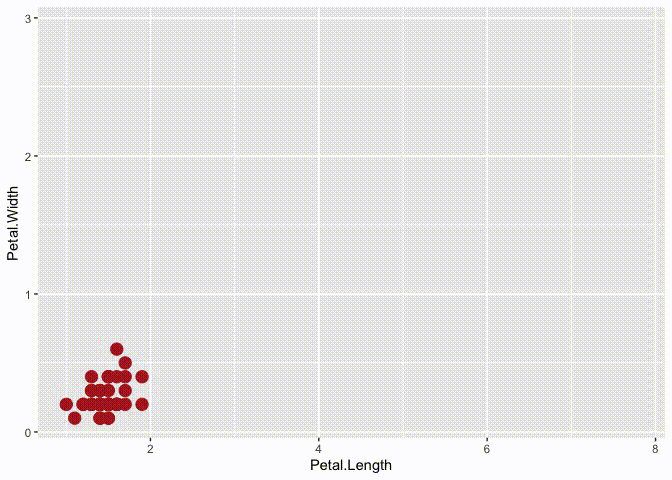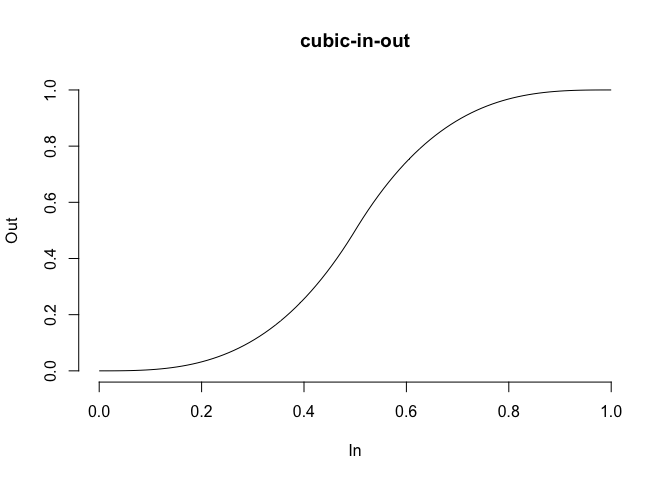# tweenr## What is this?

`tweenr` is a package for interpolating data, mainly for animations. It provides a range of functions that take data of different forms and calculate intermediary values. It supports all atomic vector types along with `factor`, `Date`, `POSIXct`, characters representing colours, and `list`. `tweenr` is used extensibly by `gganimate` to create smooth animations, but can also be used by itself to prepare data for animation in another framework.

## How do I get it?

`tweenr` is available on CRAN and can be installed with `install.packages('tweenr')`. In order to get the development version you can install it from github with `devtools`

``````#install.packages('devtools')
devtools::install_github('thomasp85/tweenr')``````

## An example

Following is an example of using the pipeable `tween_state()` function with our belowed iris data:

``````library(tweenr)
library(ggplot2)

# Prepare the data with some extra columns
iris\$col <- c('firebrick', 'forestgreen', 'steelblue')[as.integer(iris\$Species)]
iris\$size <- 4
iris\$alpha <- 1
iris <- split(iris, iris\$Species)

# Here comes tweenr
iris_tween <- iris\$setosa %>%
tween_state(iris\$versicolor, ease = 'cubic-in-out', nframes = 30) %>%
keep_state(10) %>%
tween_state(iris\$virginica, ease = 'elastic-out', nframes = 30) %>%
keep_state(10) %>%
tween_state(iris\$setosa, ease = 'quadratic-in', nframes = 30) %>%
keep_state(10)

# Animate it to show the effect
p_base <- ggplot() +
geom_point(aes(x = Petal.Length, y = Petal.Width, alpha = alpha, colour = col,
size = size)) +
scale_colour_identity() +
scale_alpha_identity() +
scale_size_identity() +
coord_cartesian(xlim = range(iris_tween\$Petal.Length),
ylim = range(iris_tween\$Petal.Width))
iris_tween <- split(iris_tween, iris_tween\$.frame)
for (d in iris_tween) {
p <- p_base %+% d
plot(p)
}``````## Other functions

Besides the `tween_state()`/`keep_state()` combo showcased above, there are a slew of other functions meant for data in different formats

`tween_components` takes a single data.frame, a vector of ids identifying recurrent elements, and a vector of timepoints for each row and interpolate each element between its specified time points.

`tween_events` takes a single data.frame where each row encodes a single unique event, along with a start, and end time and expands the data across a given number of frames.

`tween_along` takes a single data.frame along with an id and timepoint vector and calculate evenly spaced intermediary values with the possibility of keeping old values at each frame.

`tween_at` takes two data.frames or vectors along with a numeric vector giving the interpolation point between the two data.frames to calculate.

`tween_fill` fills missing values in a vector or data.frame by interpolating between previous and next non-missing elements

## Easing

In order to get smooth transitions you’d often want a non-linear interpolation. This can be achieved by using an easing function to translate the equidistant interpolation points into new ones. `tweenr` has support for a wide range of different easing functions, all of which can be previewed using `display_ease()` as here where the popular cubic-in-out is shown:

``tweenr::display_ease('cubic-in-out')``## Spatial interpolations

The purpose of `tweenr` is to interpolate values independently. If paths and polygons needs to be transitioned the `transformr` package should be used as it expands tweenr into the spatial realm